Combination of Resistors

Combination of Resistors

Resistors can be combined in two ways; series and parallel. Combination of more than one resistor is called equivalent resistor. We first look at the resistors in series;

Resistors in Series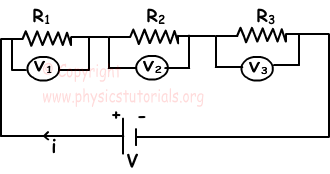a. In this types of circuit, amount of currents passing through the resistors are equal and this current comes from the battery.

i=i1=i2=i3

b. Sum of the potential differences of each resistor is equal to total potential difference of the circuit or potential difference between the ends of battery.

V=V1+V2+V3

c. If ,

V1=i1.R1

V2=i2.R2

V3=i3.R3

V=V1+V2+V3

i.Req=i1.R1=i2.R2=i3.R3

Since the currents are equal to each other;

Req=R1+R2+R3

When you add new resistors to the circuit in series, equivalent resistance of the circuit increases.

Resistors in Parallel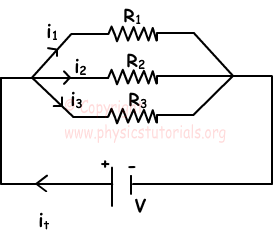a. Sum of the currents in each branch is equal to the current coming from battery.

it=i1+i2+i3

b. Since the two ends of each resistor are connected to the same points, potential differences of each resistor are equal.

V=V1=V2=V3

c.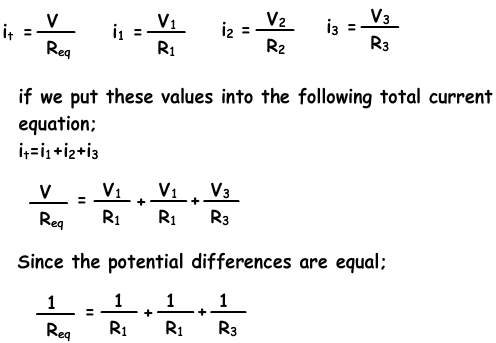When you add new resistors to the circuit in parallel, equivalent resistance of the circuit decreases.

d. If n number of resistors are combined in parallel like in the picture given below, we find the equivalent resistance of the circuit as;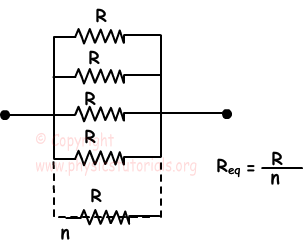Example: Find the, equivalent resistance, currents passing through each resistor and potential difference between the ends of each resistor of the circuit given below.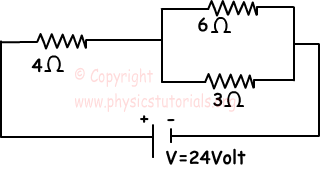Since 3Ω and 6Ω resistors are in parallel, their equivalence becomes;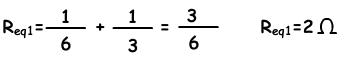Since 4Ω and Req1 resistors are in series, their equivalence becomes;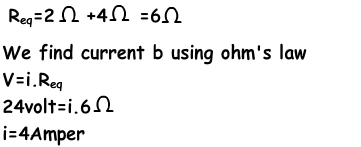Since the equivalent resistance of 3Ω and 6Ω is 2Ω, potential difference between the ends of this resistor is;

V=4A.2Ω=8volt

Currents in the resistors are;

i3=8volt/3Ω=8/3Amper

i6=8volt/6Ω=4/3Amper

i4=4Amper

Potential difference between the ends of the 4Ω resistance is;

V=i.R

V=4A.4Ω=16volt

Electric Current Exams and Solutions

Author: